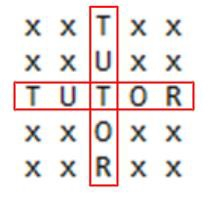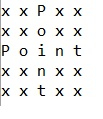# Printing string in plus ‚Äò+‚Äô pattern in the matrix in C++

Given a string str, we have to print the given string str in ‘+’ pattern in the matrix. To form plus pattern in a matrix the matrix must be a square matrix. A square matrix is that matrix which has same number of rows and column.

Like we have a string “Tutor” our task is to print the string horizontally and vertically intersecting each other from the center, and make the rest of the elements of the matrix as “x” like in the given figure −Input

str[] = {“Point”}

OutputInput

str[] = {“this”}

Output

Pattern not possible

## Approach used below is as follows to solve the problem

• Get the input.

• Check if the input is not an even length.

• Initially set the whole matrix with “x”

• Set the string in middle row and middle column

• Print the resultant matrix.

## Algorithm

Start
In function int stringcross(char str[], int n)
Step 1→ If n % 2 == 0 then,
Step 2→ Printf "Pattern not possible”
Step 3→ Else
Declare a str2[max][max]
Declare m and set as n / 2
For i = 0 and i < n and i++
For j = 0 and j < n and j++
Set str2[i][j] as 'x'
For i = 0 and i < n and i++
Set str2[i][m] as str[i]
For i = 0 and i < n and i++
Set str2[m][i] as str[i]
For i = 0 and i < n and i++
For j = 0 and j < n and j++
Print str2[i][j]
Print newline
In Function int main()
Step 1→ Declare and Initialize str[] as "TUTOR"
Step 2→ Declare and Initialize n with the size of the string
Step 3→ Call stringcross(str, n-1)
Stop

## Example

Live Demo

#include <stdio.h>
#define max 100
int stringcross(char str[], int n){
if (n % 2 == 0){
//odd length string is only possible
printf("Pattern not possible\n");
}
else {
//decalaring a 2-d character array
char str2[max][max];
int m = n / 2;
//Initially setting x for all elements
for (int i = 0; i < n; i++) {
for (int j = 0; j < n; j++) {
str2[i][j] = 'x';
}
}
//Placing the string in a manner
//a cross is formed.
for (int i = 0; i < n; i++){
//for middle columns
str2[i][m] = str[i];
}
for (int i = 0; i < n; i++){
//for middle row
str2[m][i] = str[i];
}
//printing
for (int i = 0; i < n; i++) {
for (int j = 0; j < n; j++) {
printf("%c ",str2[i][j]);
}
printf("\n");
}
}
return 0;
}
int main(){
char str[] = {"TUTOR"};
int n = sizeof(str)/sizeof(str);
stringcross(str, n-1);
return 0;
}

## Output

If run the above code it will generate the following output −